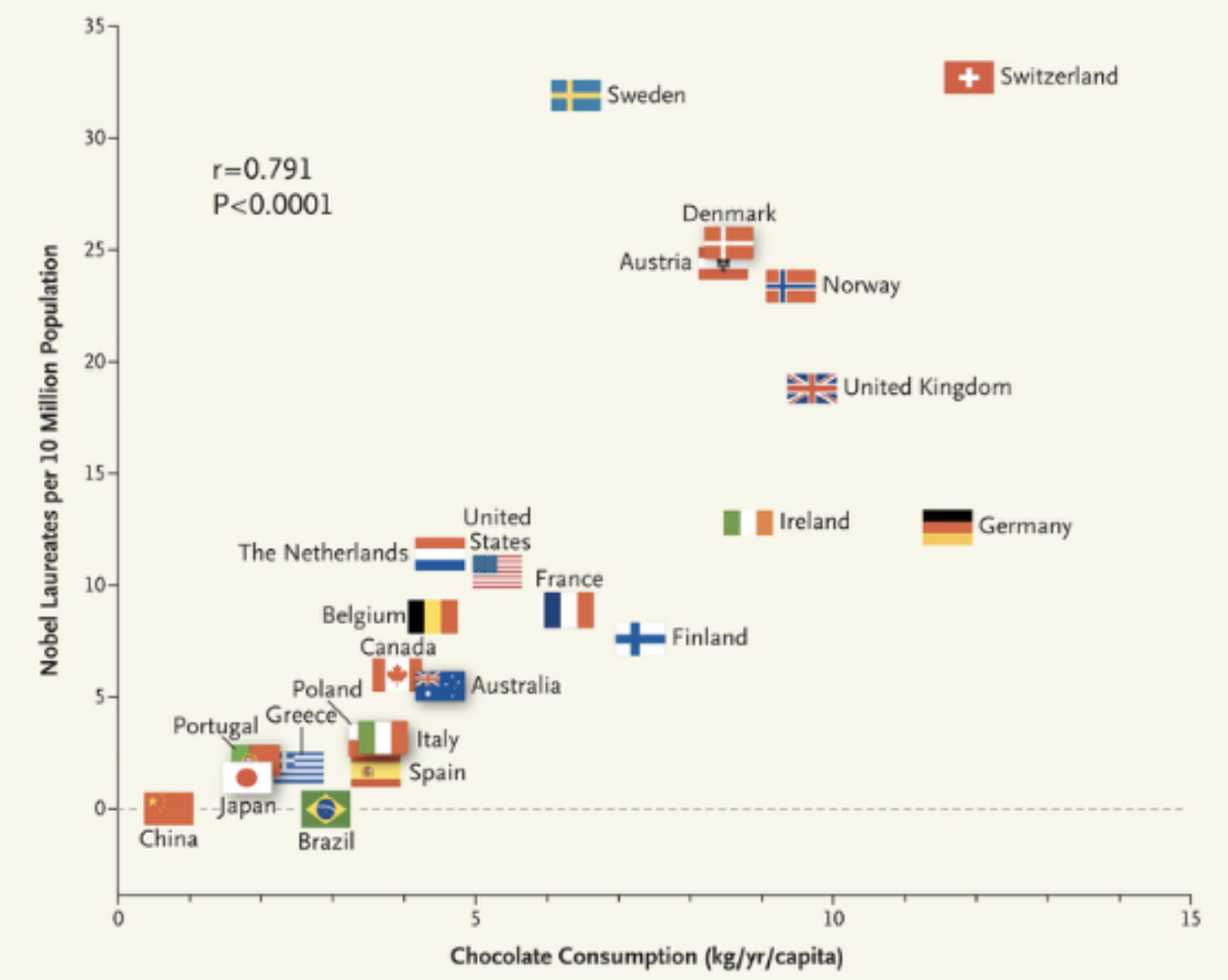# Lecture 8: Causal Discovery and Inference

Learning and inference algorithms for causal discovery.

Causation implies correlation (or dependence), but correlation does not imply causation. For example, consider the case of chocolate consumption vs. the number of Nobel Laureates in the country:Chocolate Consumption vs. # of Nobel Laureates

From this plot, we can see that they are highly correlated. But it is absurd to say that if we want to increase the number of Nobel Laureates in a country, we need to eat more chocolate. This is where the idea of causality comes in. They are correlated, but chocolate consumption is not the cause of Nobel Laureates. The following equations capture this difference mathematically:

$X$ and $Y$ are dependent if and only if $\exists x_1$ different than $x_2$ such that:

$P(Y \mid X=x_{1}) \text{ different than } P(Y \mid X=x_2)$

$X$ is a cause of $Y$ if and only if $\exists x_1$ different than $x_2$ such that:

$P(Y \mid do(X=x_1) \text{ different than } P(Y \mid X=x_2)$

The definition requires just a single pair of distinct $x_1, x_2$ to have different conditional distributions of $Y$. $Y$ may have the same conditional distribution over a range of $X$ values, but if we find even a single pair for which the conditional distribution is different, $X$ and $Y$ are dependent. The same goes for causation.

The causation definition is circular in the sense that, if we don’t know the causal relation of the other variables, we can’t define the intervention of $X$ and thus can’t find the causal relation of $X$ and $Y$. So in order to define one causal relation, we need all other causal relations.

## Causal Thinking

### Simpson’s Paradox

Example 1: Suppose there are two types of patients, one with small kidney stones and the other with large kidney stones. There are two types of treatments to choose from, A and B. From the data we can see that, if we consider the patient categories separately, treatment A has a higher recovery rate in both cases. But if we combine the categories of patients, we see that the treatment B has a higher recovery rate 83% (289/350). So, as a doctor, which treatment would you suggest to a patient?

Example 2: We plot cholesterol levels vs. the amount of exercise for people of different age groups. For each age group, we see that the more you exercise, the less cholesterol you have. But if we combine all the plots (of different age groups), we see the opposite result: the more you exercise, the more cholesterol you have.

In both examples, there was a common cause between the quantities we were comparing, and due to this common cause, the relationship between the quantities can be arbitrary. We should fix the value of the common cause, and then see the relation between the quantities we want to compare.

### Strange Dependence

Example: If we go back 50 years, we observe that female college students score higher on IQ tests than male students on average. Gender and IQ are two very different quantities, and we can safely assume that they are independent. But given a common cause, like say going to college, now they become dependent. A simple way to understand this is as follows: Two independent variables $X$ and $Y$ become dependent if we condition on the sum of $X$ and $Y$.